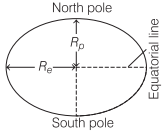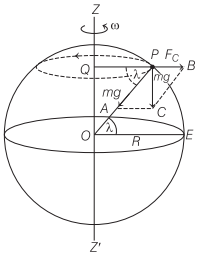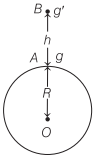# Factors Affecting Acceleration Due to Gravity | Physics – Gravitation

## Factors Affecting Acceleration Due to Gravity | Physics – Gravitation

We are giving a detailed and clear sheet on all Physics Notes that are very useful to understand the Basic Physics Concepts.

Factors Affecting Acceleration Due to Gravity:

(i) Shape of Earth:
Acceleration due to gravity g α $$\frac{1}{R^{2}}$$.
Earth is elliptical in shape. Its diameter at poles is approximately 42 km less than its diameter at equator.
Therefore, g is minimum at equator and maximum at poles.(ii) Rotation of Earth about Its Own Axis:
If ω is the angular velocity of rotation of earth about its own axis, then acceleration due to gravity at a place having latitude λ is given by
$$g^{\prime}$$ = g – Rω² cos² λAt poles λ = 90° and $$g^{\prime}$$ = g

Therefore, there is no effect of rotation of earth about its own axis at poles.
At equator λ = 0° and g’ = g – Rω²
The value of g is minimum at equator.
If earth stops its rotation about its own axis, then g will remain unchanged at poles but increases by Rω² at equator.

(iii) Effect of Altitude:
The value of g at height h from earth’s surface$$g^{\prime}=\frac{g}{\left(1+\frac{h}{R}\right)^{2}}$$

For h << R
$$g^{\prime}=g\left(1-\frac{2 h}{R}\right)$$
Therefore, g decreases with altitude.

(iv) Effect of Depth:
The value of g at depth h from earth’s surface$$g^{\prime}=g\left(1-\frac{h}{R}\right)$$

Therefore, g decreases with depth from earth’s surface. The value of g becomes zero at earth’s centre.

Gravitation:
Have you ever thought, when we throw a ball above the ground level, why it returns back to the ground. It’s because of gravity. When a ball is thrown above the ground in the opposite direction, a gravitational force acts on it which pulls it downwards and makes it fall. This phenomena is called gravitation.

Learn relation between gravitational field and potential field, Kepler’s law of planetary, weightlessness of objects in absence of gravitation, etc.